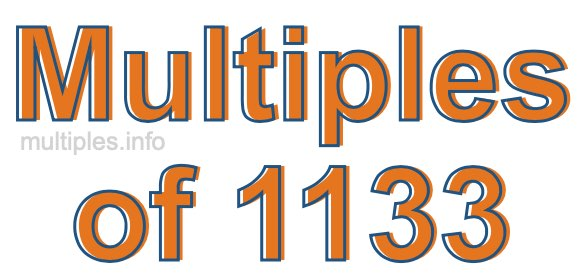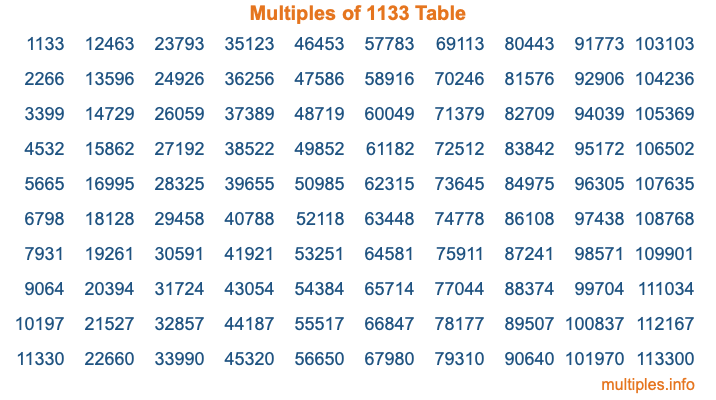Multiples of 1133Welcome to the Multiples of 1133 page. Here we will first teach you everything you will ever need to know about the multiples of 1133, and then give you a study guide summary of everything we taught you to make sure you remember it all. Use this page to look up facts and learn information about the multiples of 1133. This page will make you a multiples of one thousand one hundred thirty-three expert!

Definition of Multiples of 1133
Multiples of 1133 are all the numbers that when divided by 1133 equal an integer. Each of the multiples of 1133 are called a multiple. A multiple of 1133 is created by multiplying 1133 by an integer.

Therefore, to create a list of multiples of 1133, you start with 1 multiplied by 1133, then 2 multiplied by 1133, then 3 multiplied by 1133, and so on for as long as you want. Thus, the list of the first five multiples of 1133 is 1133, 2266, 3399, 4532, and 5665. To see a larger list of multiples of 1133, see the printable image of Multiples of 1133 further down on this page. We also have a category where you can choose any nth multiple of 1133.

Multiples of 1133 Checker
The Multiples of 1133 Checker below checks to see if any number of your choice is a multiple of 1133. In other words, it checks to see if there is any number (integer) that when multiplied by 1133 will equal your number. To do that, we divide your number by 1133. If the the quotient is an integer, then your number is a multiple of 1133.

Is  a multiple of 1133?

Least Common Multiple of 1133 and ...
A Least Common Multiple (LCM) is the lowest multiple that two or more numbers have in common. This is also called the smallest common multiple or lowest common multiple and is useful to know when you are adding our subtracting fractions. Enter one or more numbers below (1133 is already entered) to find the LCM.

Check out our LCM Calculator if you need more details about the Least Common Multiple or if you need the LCM for different numbers for adding and subtraction fractions.

nth Multiple of 1133
As we stated above, 1133 is the first multiple of 1133, 2266 is the second multiple of 1133, 3399 is the third multiple of 1133, and so on. Enter a number below to find the nth multiple of 1133.

th multiple of 1133

Multiples of 1133 vs Factors of 1133
1133 is a multiple of 1133 and a factor of 1133, but that is where the similarities end. All postive multiples of 1133 are 1133 or greater than 1133. All positive factors of 1133 are 1133 or less than 1133.

Below is the beginning list of multiples of 1133 and the factors of 1133 so you can compare:

Multiples of 1133: 1133, 2266, 3399, 4532, 5665, etc.

Factors of 1133: 1, 11, 103, 1133

As you can see, the multiples of 1133 are all the numbers that you can divide by 1133 to get a whole number. The factors of 1133, on the other hand, are all the whole numbers that you can multiply by another whole number to get 1133.

It's also interesting to note that if a number (x) is a factor of 1133, then 1133 will also be a multiple of that number (x).

Multiples of 1133 vs Divisors of 1133
The divisors of 1133 are all the integers that 1133 can be divided by evenly. Below is a list of the divisors of 1133.

Divisors of 1133: 1, 11, 103, 1133

The interesting thing to note here is that if you take any multiple of 1133 and divide it by a divisor of 1133, you will see that the quotient is an integer.

Multiples of 1133 Table
Below is an image of the first 100 multiples of 1133 in a table. The table is in chronological order, column by column. The first column has the first ten multiples of 1133, the second column has the next ten multiples of 1133, and so on.The Multiples of 1133 Table is also referred to as the 1133 Times Table or Times Table of 1133. You are welcome to print out our table for your studies.

Negative Multiples of 1133
Although not often discussed or needed in math, it is worth mentioning that you can make a list of negative multiples of 1133 by multiplying 1133 by -1, then by -2, then by -3, and so on, to get the following list of negative multiples of 1133:

-1133, -2266, -3399, -4532, -5665, etc.

Multiples of 1133 Summary
Below is a summary of important Multiples of 1133 facts that we have discussed on this page. To retain the knowledge on this page, we recommend that you read through the summary and explain to yourself or a study partner why they hold true.

There are an infinite number of multiples of 1133.

A multiple of 1133 divided by 1133 will equal a whole number.

1133 divided by a factor of 1133 equals a divisor of 1133.

The nth multiple of 1133 is n times 1133.

The largest factor of 1133 is equal to the first positive multiple of 1133.

1133 is a multiple of every factor of 1133.

1133 is a multiple of 1133.

A multiple of 1133 divided by a divisor of 1133 equals an integer.

1133 divided by a divisor of 1133 equals a factor of 1133.

Any integer times 1133 will equal a multiple of 1133.

Multiples of a Number
Here you can get the multiples of another number, all with the same attention to detail as we did for multiples of 1133 on this page.

Multiples of
Multiples of 1134
Did you find our page about multiples of one thousand one hundred thirty-three educational? Do you want more knowledge? Check out the multiples of the next number on our list!